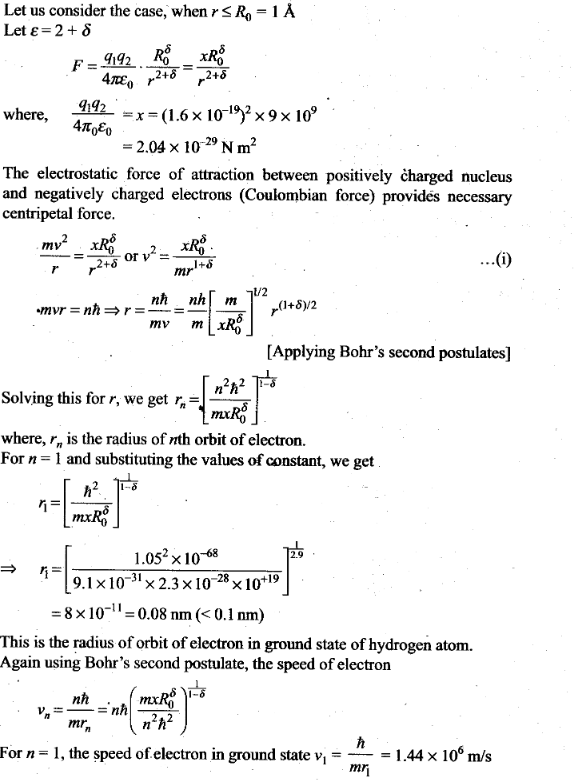# Atoms NCERT Exemplar Problems Solutions Physics

0
2088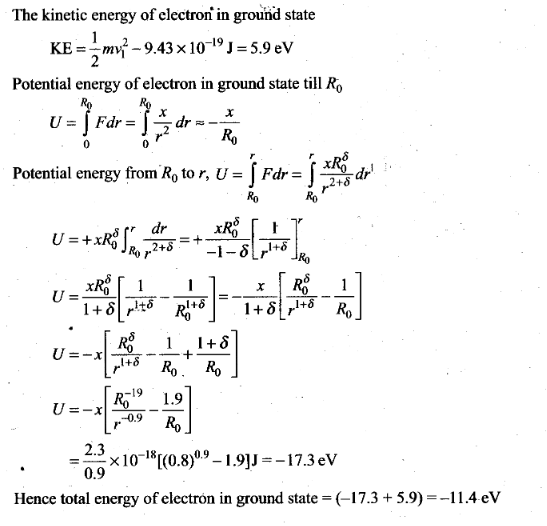## NCERT Exemplar Problems Class 12 Physics Chapter 12 Atoms

Multiple Choice Questions (MCQs)

Question 1. Taking the Bohr radius as a0= 53 pm, the radius of Li++ ion in its ground state, on the basis of Bohr’s model, will be about
(a) 53 pm (b) 27 pm (c) 18 pm (d) 13 pm
Solution: (c)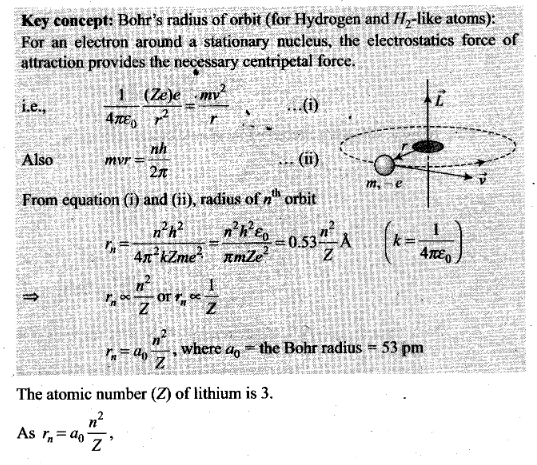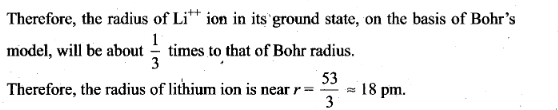Question 2. The binding energy of a H-atom, considering an electron moving around a fixed nuclei (proton), is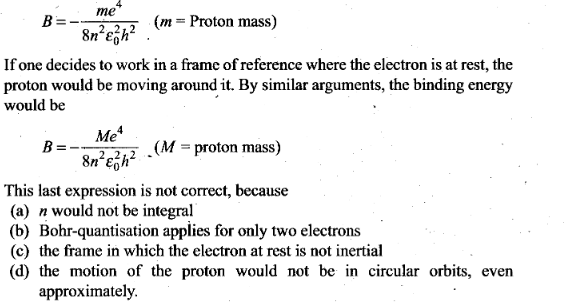Solution: (c) In a hydrogen atom, electron revolving around a fixed proton nucleus have some centripetal acceleration. Therefore its frame of reference is non- inertial. If the frame of reference, where the electron is at rest, the given expression is not true as it forms the non-inertial frame of reference.

Question 3. The simple Bohr model cannot be directly applied to calculate the energy levels of an atom with man electrons. This is because
(a) of the electrons not being subject to a central force
(b) of the electrons colliding with each other
(c) of screening effects
(d) the force between the nucleus and an electron will no longer be given by Coulomb’s law
Solution: (a) The simple Bohr model cannot be directly applied to calculate the energy levels of an atom with many electrons because when we derive the formula for radius/energy levels etc, we make the assumption that centripetal force is provided only by electrostatic force of attraction by the nucleus. Hence, this will only work for single electron atoms. In multi-electron atoms, there will also be repulsion due to other electrons. The simple Bohr model cannot be directly applied to calculate the energy levels of an atom with many electrons.

Question 4. For the ground state, the electron in the H-atom has an angular .momentum = h, according to the simple Bohr model. Angular momentum is a vector and hence there will be infinitely many orbits with the vector pointing in all possible directions. In actuality, this is not true,
(a) because Bohr model gives incorrect values of angular momentum
(b) because only one of these Would Have a minimum energy
(c) angular momentum must be in the direction of spin of electron
(d) because electrons go around only in horizontal orbits
Solution: (a)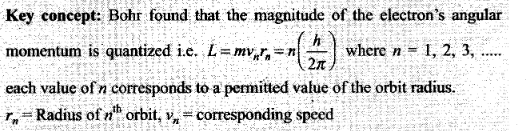Bohr’s model gives only the magnitude of angular momentum. The angular momentum is a vector quantity. Hence we cannot express angular momentum completely by Bohr model. Hence it does not give correct values of angular momentum of revolving electron.

Question 5. 02 molecule consists of two oxygen atoms. In the molecule, nuclear force between the nuclei of the two atoms
(a) is not important because nuclear forces are short-ranged
(b) is as important as electrostatic force for binding the two atoms
(c) cancels the repulsive electrostatic force between the nuclei
(d) is not important because oxygen nucleus have equal number of neutrons and protons
Solution: (a)
Key concept: Forces that keep the nucleons bound in the nucleus are called nuclear forces.
• Nuclear forces are short range forces. These do not exist at large distances greater than 10~15 m.
• Nuclear forces are the strongest forces in nature.
• These are attractive force and causes stability of the nucleus.
• These forces are charge independent.
• Nuclear forces arc non-central force.
The nuclear binding force has to dominate over the Coulomb repulsive force between protons inside the nucleus. The nuclear force between two nucleons falls rapidly to zero as their distance is more than a few femtometres. .
In O2 molecule which consists of two oxygen atoms molecules, nuclear force between the nuclei of the two atoms is not important because nuclear forces are short-ranged and act inside the nucleus only.

Question 6. Two H atoms in the ground state collide inelastically. The maximum amount by which their combined kinetic energy is reduced is
(a) 10.20 eV (b) 20.40eV (c) 13.6 eV
Solution: (a)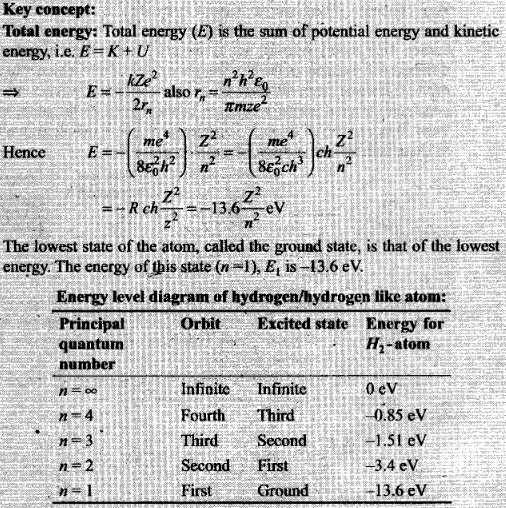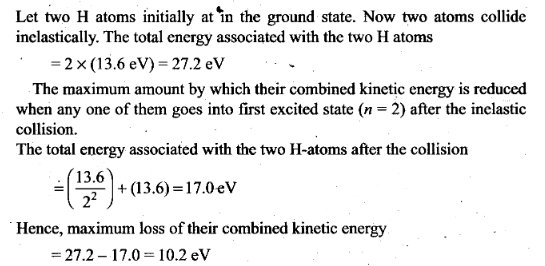Question 7. A set of atoms in an excited state decays
(a) in general to any of the states with lower energy
(b) into a lower state only when excited by an external electric field
(c) all together simultaneously into a lower state
(d) to emit photons only when they collide .
Solution: (a)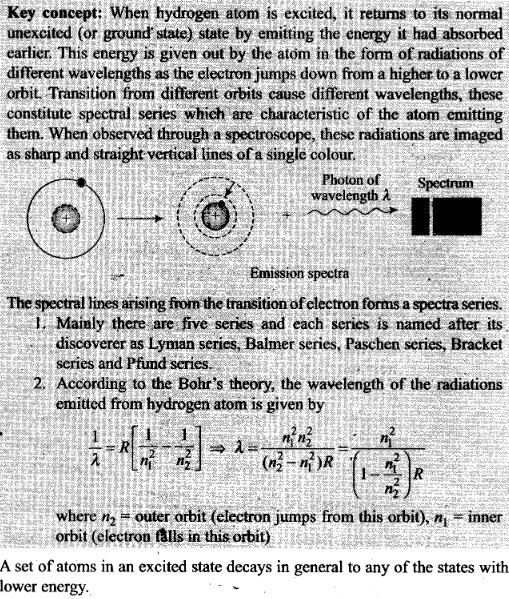One or More Than One Correct Answer Type

Question 8. An ionised H-molecule consists of an electron and two protons. The protons are separated by a small distance of the order of angstrom. In the ground state,
(a) the electron would not move in circular orbits
(b) the energy would be (2)4 times that of a H-atom
(c) the electrons, orbit would go around the protons
(d) the molecule will soon decay in a proton and a H-atom
Solution: (a, c) In a hydrogen atom, electron revolves around a fixed proton nucleus in circular path. This can be explained by Bohr model. But in case of ionised H-molecule which consists of two protons in nucleus and where protons are separated by a small distance of the order of angstrom, cannot be explained by Bohr model. Hence in this case the ground state the electron would not move in circular orbits, the electrons orbit would go around the protons.

Question 9. Consider aiming a beam of free electrons towards free protons. When-they scatter, an electron and a proton cannot combine to produce a H-atom.
(a) Because of energy conservation
(b) Without simultaneously releasing energy in the form of radiation
(c) Because of momentum conservation
(d) Because of angular momentum conservation
Solution: (a, b) A moving electron and proton cannot combine to produce a H-atom because of energy conservation and without simultaneously releasing energy in the form of radiation.

Question 10. The Bohr model for the spectra of a H-atom
(a) will not be applicable to hydrogen in the molecular form
(b) will not be applicable as it is for a He-atom
(c) is valid only at room temperature
(d) predicts continuous as well as discrete spectral lines
Solution: (a, b) Bohr proposed a-model for hydrogen atom which is also applicable for some lighter atoms in which a single electron revolves around a stationary nucleus of positive charge Ze (called hydrogen like atom, e.g.: H, He+, Li+2, Na+1 etc). It is not applicable to hydrogen in the molecular form and also, it will not be applicable as it is for a He-atom.

Question 11. The Balmer series for the H-atom can be observed
(a) if we measure the frequencies of light emitted when an excited atom falls to the ground state
(b) if we measure the frequencies of light emitted due to transitions between
excited states and the first excited state
(c) in any transition in a H-atom .
(d) as a sequence of frequencies with the higher frequencies getting closely
Solution: (b, d)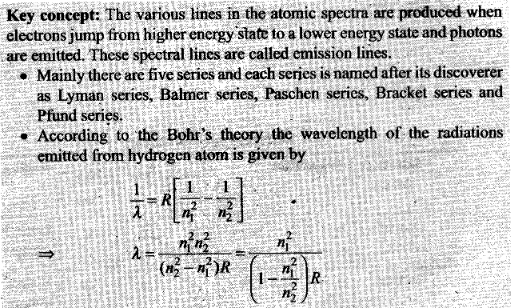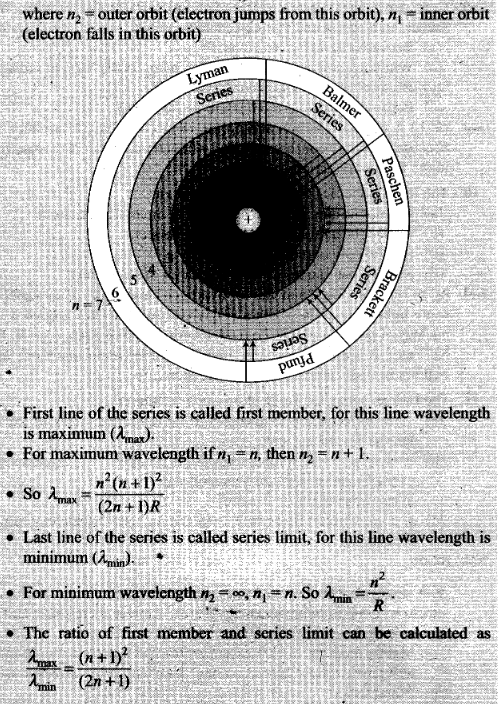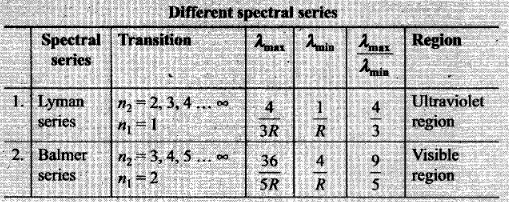From above discussion we can say Balmer series for the H-atom can be observed if we measure the frequencies of light emitted due to transitions between higher excited states and the first excited state and as a sequence of frequencies with the’higher frequencies getting closely packed.

Question 12.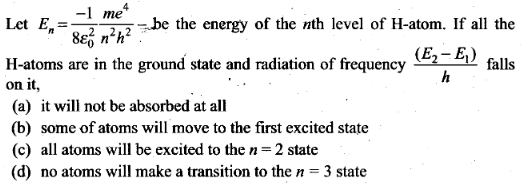Solution: (b, d) Let E2 and E1 be the energy corresponding to n = 2 and n = 1 respectively. If radiation of energy ∆E = (E2 – E1) = hf incident on a sample where all the H-atoms are in the ground state, according to Bohr model some of the atoms will move to the first excited state. As this energy is not sufficient for transition from n = 1 to n =3, hence no atoms will make a transition to the n = 3 state.

Question 13. The simple Bohr model is not applicable to He4 atom because
(a) He4 is an inert gas
(b) He4 has neutrons in the nucleus
(c) He4 has one more electron
(d) electrons are not subject to central forces
Solution: (c, d)
Key concept: Bohr proposed a model for hydrogen atom which is also applicable for some lighter atoms in which a single electron revolves around a stationary nucleus of positive charge Ze (called hydrogen like atom). It is valid only for one electron atoms, e.g. : H, He+, Li+2, Na+1 etc.
The Bohr model is not applicable to He4, as it has one more electron and electrons are not subjected to central forces.

Question 14. The mass of a H-atom is less than the sum of the masses of a proton and electron. Why is this?
Solution: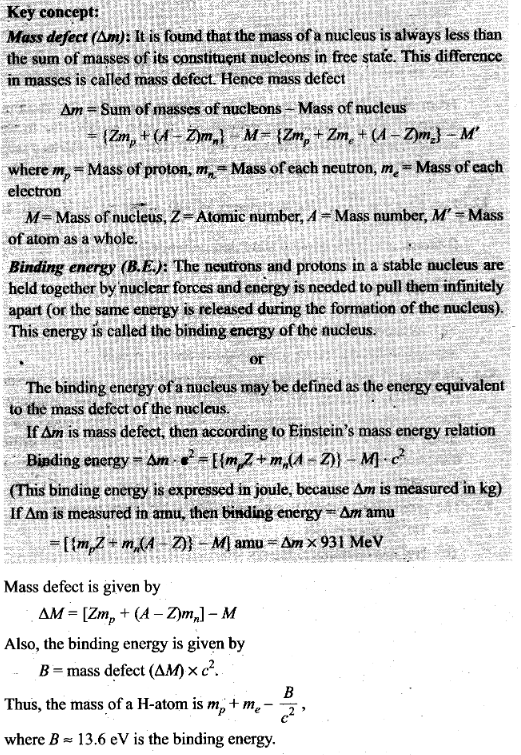Question 15. Imagine removing one electron from He4 and He3. Their energy levels, as worked out on the basis of Bohr model will be very close. Explain why.
Solution: Bohr model is applicable for hydrogen atom-and some lighter atoms in which a single electron revolves around a stationary nucleus of positive charge Ze (called hydrogen like atom). If we remove one electron from He4 and He3, atoms contain one electron and becomes hydrogen like atoms. Now we can apply Bohr model to define the energy levels.

Question 16. When an electron falls from a higher energy to a lower energy level, the
difference in the energies appears in the form of electromagnetic radiation. Why cannot it be emitted as other forms of energy? .
Solution: The electrons are charged particles. When an electron falls from a higher energy to a lower energy level, it accelerates. We know accelerating charged particle radiates energy in the form of electromagnetic radiation.

Question 17. Would the Bohr formula for the H-atom remain unchanged if proton had a charge (+ 4/3)e and electron a charge (-3/4)e, where e = 1.6 x 10-19 C. Give reasons for your answer.
Solution: According to Bohr, for an electron around a stationary nucleus the electrostatics force of attraction provides the necessary centripetal force.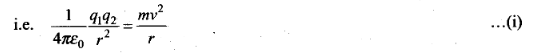Hence the magnitude of electrostatic force F ∞ q1 x q2
If proton had a charge (+4/3)e and electron a charge (-3/4)e, then the Bohr formula for the H-atom remains same, since the Bohr formula involves only the product of the charges which remain constant for given values of charges.

Question 18. Consider two different hydrogen atoms. The electron in each atom is in an excited state. Is it possible for the electrons to have different energies but the same orbital angular momentum according to the Bohr model?
Solution: Bohr postulated that an electron in an atom can move around the nucleus in a certain circular stable orbits without emitting radiations.
Bohr found that the magnitude of the electron’s angular momentum is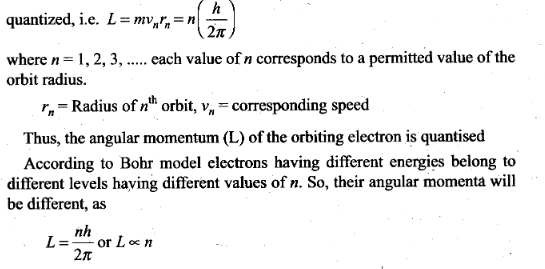Question 19. Positronium is just like a H-atom with the proton replaced by the positively charged anti-particle of the electron (called the positron which is as massive as the electron). What would be the ground state energy of positronium?
Solution:
Key concept: Positronium (Ps) is a system consisting of an electron and its anti-particle a positron, bound together into an exotic atom, specifically anonium. The system is unstable: the two particles annihilate each other to predominantly produce two or three gamma-rays, depending on the relative spin states. The orbit and energy levels of the two particles arc similar to that of the hydrogen atom (which is a bound slate of a proton and an electron). I lowever, because of the reduced mass, the frequencies of the spectral lines are less than half of the corresponding hydrogen lines.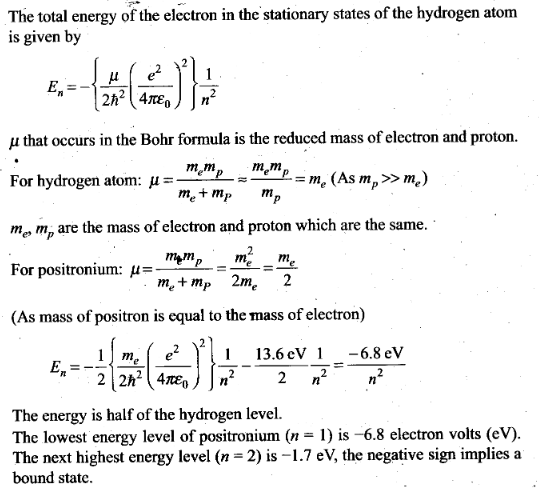Question 20. Assume that there is no repulsive force between the electrons in an atom but the force between positive and negative charges is given by Coulomb’s law as usual. Under such circumstances, calculate the ground state energy of a He-atom.
Solution: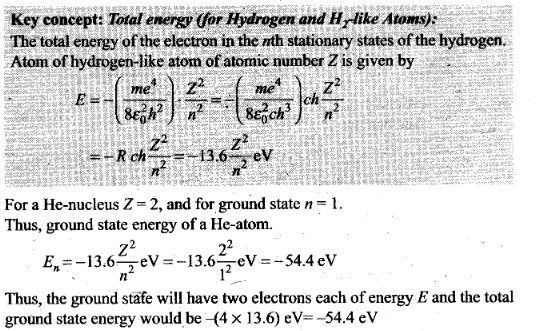Question 21. Using Bohr model, calculate the electric current created by the electron when the H-atom is in the ground state.
Solution: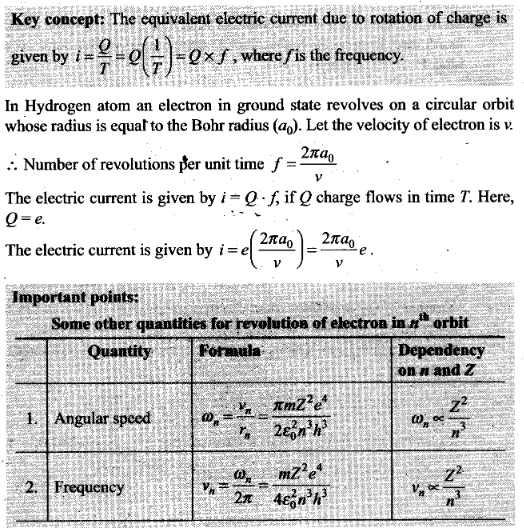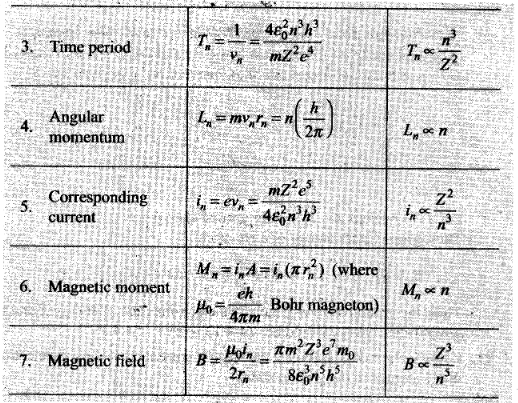Question 22. Show that the first few frequencies of light that is emitted when electrons fall to nth level from levels higher than n, are approximate harmonics (i.e., in the ratio 1:2:3 …) when n >> 1.
Solution: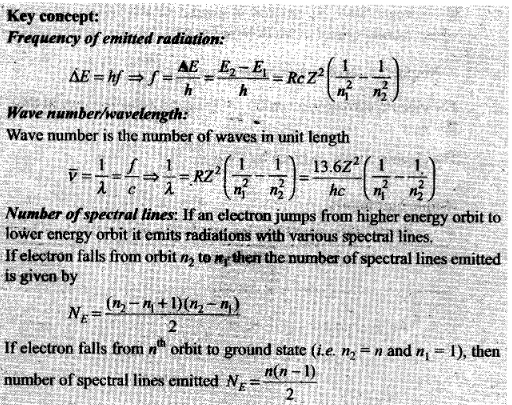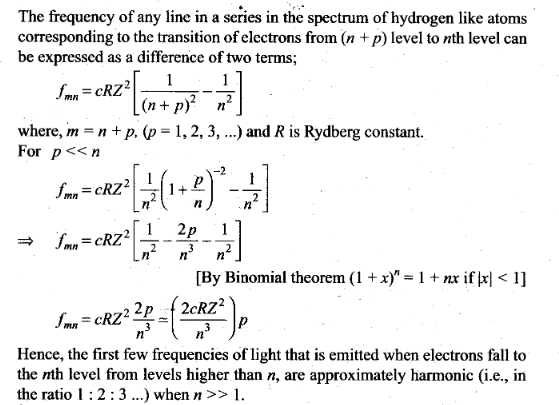Question 23. What is the minimum energy that must be given to a H-atom in ground state so that it can emit an Hґ Line in Balmer series? If the angular momentum of the system is conserved, what would be the angular momentum of such Hґ photon?
Solution: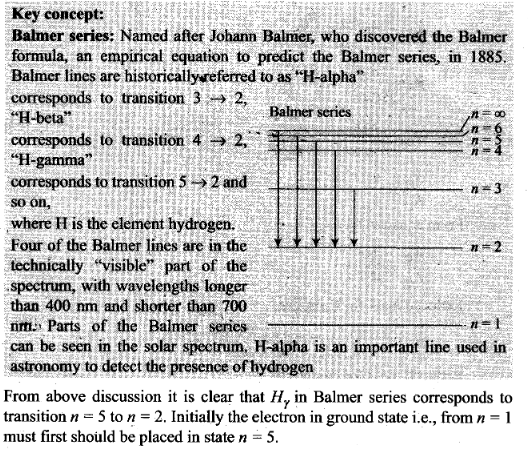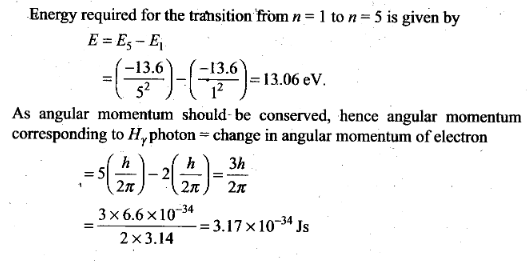Question 24. The first four spectral in the Lyman series of a H-atom are λ= 1218 Å, 1028 Å, 974.3 Å and 951.4 Å. If instead of Hydrogen, we consider deuterium, calculate the shift in the wavelength of these lines.
Solution: Let µH and µD are the reduced masses of electron for hydrogen and deuterium respectively.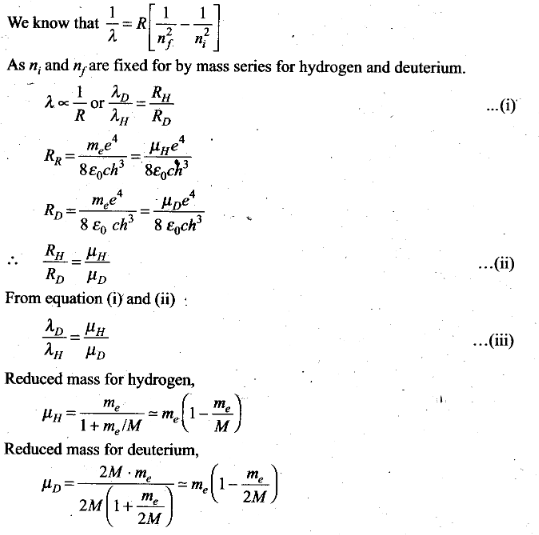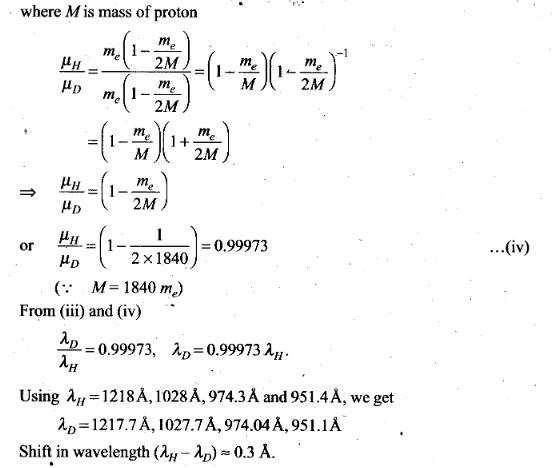Question 25. Deutrium was discovered in 1932 by Harold Urey by measuring the small change in wavelength for a particular transition in 1H and 2H. This is because, the wavelength of transition depend to a certain extent on the nuclear mass. If nuclear motion is taken into account, then the electrons and nucleus revolve around their common centre bf mass.
Such a system is equivalent to a single particle with a reduced mass µ, revolving around the nucleus at a distance equal to the electron-nucleus separation. Here µ=meMI(me + M), wfiere M is the nuclear mass and me is the electronic mass. Estimate the percentage difference in wavelength for the 1st line of the Lyman series in ‘H and 2H
(Mass of 1H nucleus is 1.6725 x 10-27 kg, mass of 2H nucleus is 3.3374 x 10-27 kg, Mass of electron = 9.109 x 10-31 kg.)
Solution: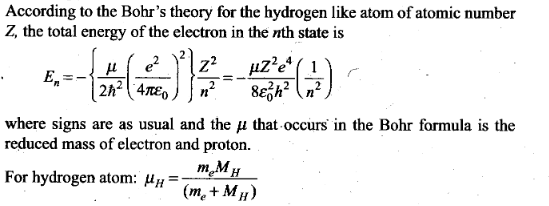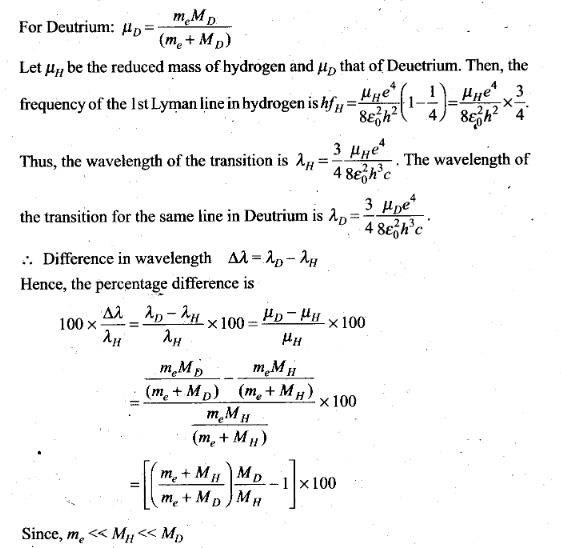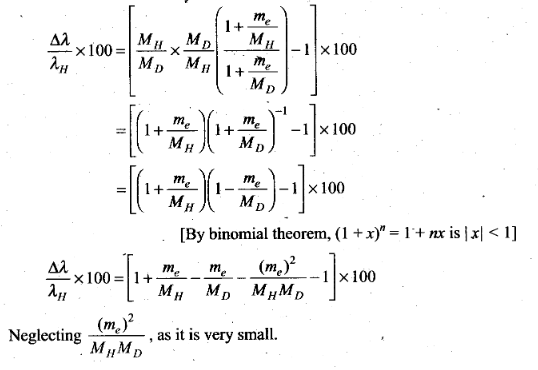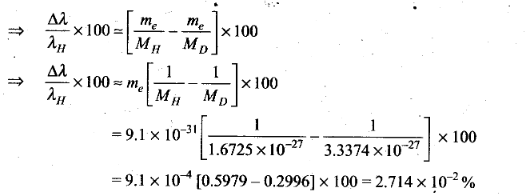Question 26. If a proton had a radius R and the charge was uniformly distributed, calculate using Bohr theory, the ground state energy of a H-atom when (i) R = 0.1 Å and (ii) R = 10 Å .
Solution: In a H-atom in ground state, electron revolves round the point-size proton in a circular orbit of radius rB (Bohr’s radius).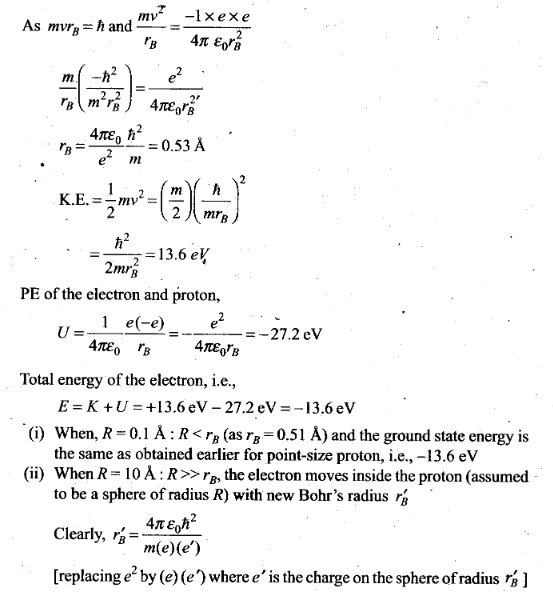Question 27. In the Auger process, an atom maizes a transition to a lower state without emitting a photon. The excess energy is transferred to an outer electron which may be ejected by the atom (This is called an Auger electron). Assuming the nucleus to be massive, calculate the kinetic energy of an n = 4 Auger electron emitted by Chromium by absorbing the energy from a n – 2 to n = 1 transition.
Solution:
Key concept:
Auger Effect: The Auger effect is a process by which electrons with characteristic energies are ejected from atoms in response to a downward transition by another electron in the atom. In Auger spectroscopy, the vacancy is produced by bombardment with high energy electrons, but the Auger effect can occur if the vacancy is produced by other interactions. It is observed as one of the methods of electron rearrangement after electron capture into the nucleus.
If an inner shell electron is removed from an atom, an electron from a higher level will quickly make the transition downward to fill the vacancy. Sometimes this transition will be accompanied by an emitted photon whose quantum energy matches the energy gap between the upper and lower level. Since for heavy atoms this quantum energy will be in the x-ray region, it is commonly called x.-ray fluorescence. This emission process for lighter atoms and outer electrons gives rise to line spectra.
In other cases, the energy released by the downward transition is given to one of the outer electrons instead of to a photon, and this electron is then ejected from the atom with an energy equal to. the energy lost by the electron which made the downward transition minus the binding energy of the electron that is ejected from the atom. Though more involved in interpretation than optical spectra, the analysis of the energy spectrum of these emitted electrons does give information about die atomic energy levels. The Auger effect bears some resemblance to internal conversion of the nucleus, which also ejects an electron
Sometimes an upper election drops to fill the vacancy, emitting a photon.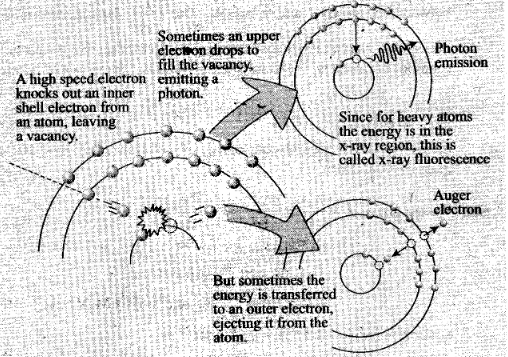As the nucleus is massive, recoil momentum of the atom may be neglected and the entire energy of the transition may be considered transferred to the Auger electron. As there is a single valence electron in Cr, the energy states may be thought of as given by the Bohr model.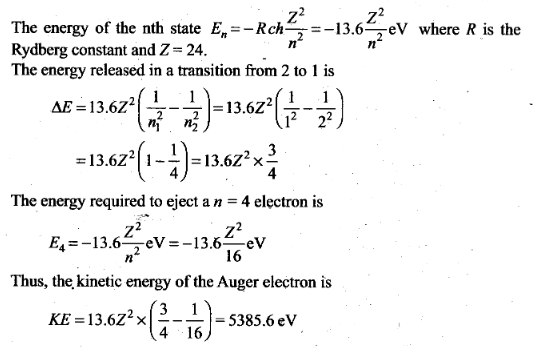Question 28.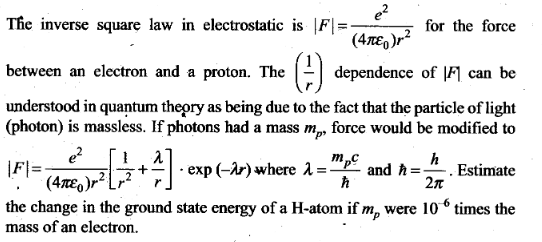Solution: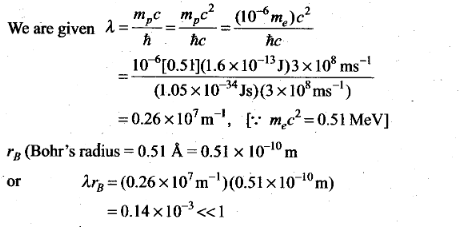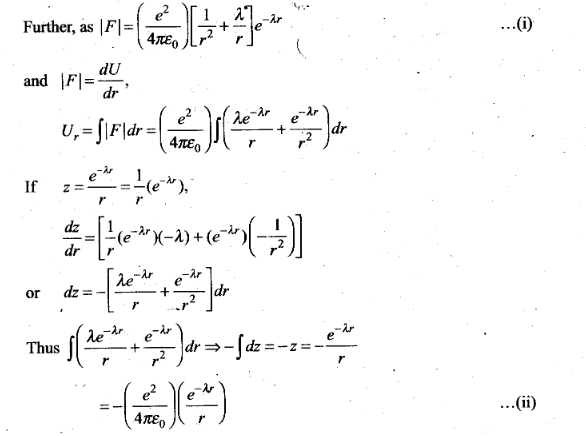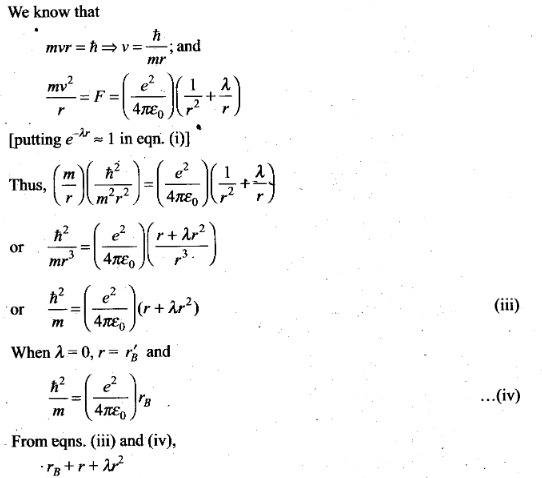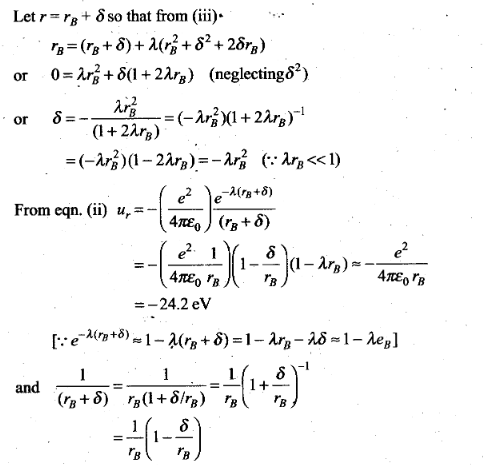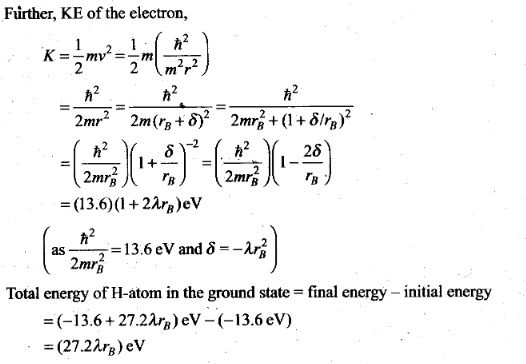Question 29. The Bohr model for the H-atom relies on the Coulomb ’s law of electrostatics. Coulomb’s law has not directly been verified for very
short distances of the order of angstroms. Supposing Coulomb’s law between two opposite charge +q1, – q2 modified to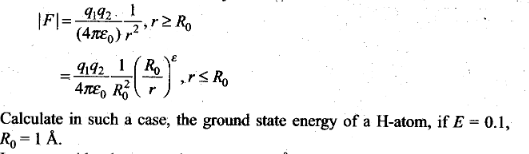Solution: